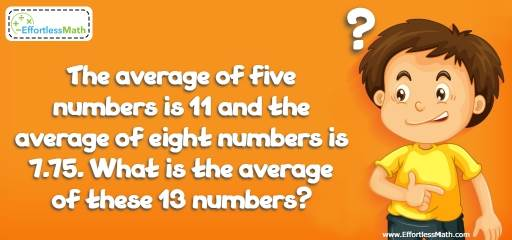# Ratio, Proportion and Percentages Puzzle -Critical Thinking 9

Math puzzles are a great way to engage students in developing critical thinking skills. This math puzzle is an educational activity for practicing children's math skills.## Challenge:

The average of five numbers is 11 and the average of eight numbers is 7.75. What is the average of these 13 numbers?

### The Absolute Best Book to Challenge Your Smart Student!

The average of five numbers is 11. Therefore, their sum is:
5 × 11 = 55
The average of eight numbers is 7.75. Therefore, their sum is:
8 × 7.75 = 62
The sum of all 13 numbers is 55 + 62 = 117
Therefore, the average of these 13 numbers is:
117 ÷ 13 = 9

The Absolute Best Books to Ace Algebra

### What people say about "Ratio, Proportion and Percentages Puzzle -Critical Thinking 9 - Effortless Math: We Help Students Learn to LOVE Mathematics"?

No one replied yet.

X
45% OFF

Limited time only!

Save Over 45%

SAVE $40 It was$89.99 now it is \$49.99